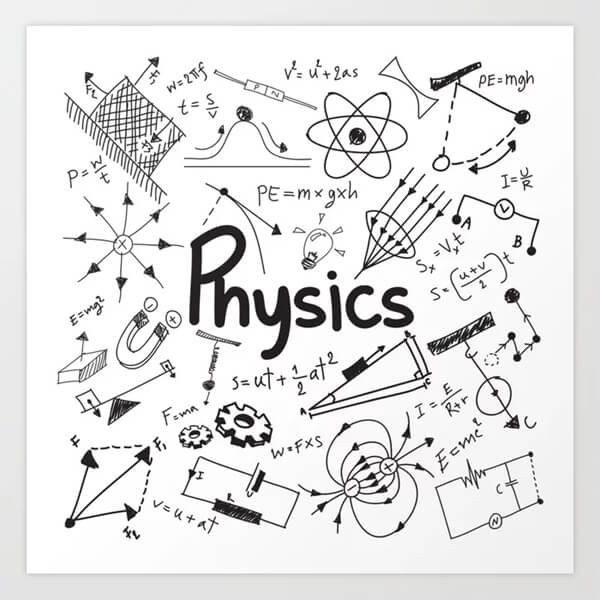Syllabus of Physics Class 11 for NEET 2020 - Study24x7Default error msg

New to Study24x7 ?

# Syllabus of Physics Class 11 for NEET 2020

Updated on 13 July 2021NEET Preparation
Updated on 13 July 2021NEET full form is National Eligibility Cum Entrance Test which is conducted by CBSE for undergraduate admission in MBBS and BDS courses. Questions that come for this test are equally distributed from Class 11 and 12 studies.

Also, Physics in NEET is considered one of the toughest subjects for many Biology based students. Therefore, for a good score in Physics,

you must know chapter-wise weightage for NEET. Though the NEET syllabus does not mention the weightage through the previous year’s paper, you can gauge the weightage of a particular chapter. This is the way you can streamline and focus more on the NEET preparation.

Based on the previous year’s paper, here are some of the important chapters or topics which has more probability of high weightage in NEET 2020.Kinematics: Mechanics overall is the most important area for Physics NEET paper. Kinematics comes under Mechanics and deals with the study of motion. Average number of questions from this chapter is expected to be 2 that is 4% of the total questions of Mechanics.

Though the kinematics equation looks easy students must not leave practicing as these are the areas where one can answer correctly and increase the score considerably. Do not ignore the examples and numerical for NCERT textbooks.

Try to consider real-life phenomena and relate problems. This would help you to draw diagrams and solve numerically. Be extremely hands-on with the vectors numerical.

Also, based on projectile motion practice numerical related to constrained motion or circular motion. This has importance not only in NEET but also in all types of All India JEE.

Laws of Motion: Learn the equation related to laws of motion and their use. Around 3 questions are expected from this part that is 7% of the total questions from the Mechanics area. Practice as many numerical you can from this part.

Work, Energy, and Power: ‘Energy’ is a buzzword for most physics modules. Work, Energy, and Power is a topic that comes under Mechanics and the average number of questions expected from this topic is 2 i.e 4% of the total questions of Mechanics.

Work energy theorem, equation related to gravitational potential energy are some of the areas that you need to concentrate on as most of the questions appear from this part. Power is a smaller part so you can expect a theoretical question.

However, the students must also be thorough in the theory apart from numerical. This is because at times real-life theoretical questions might become difficult to relate if you are not thorough.

The motion of System of Particles and Rigid Body: You can expect around 3 questions from this module of Mechanics as well.

of mass, Angular Momentum, and Rotational motion of various objects. However, equations are very important to answer theoretical as well as numerical questions from this topic.

Apart from the NCERT textbook, it is also advisable to practice from previous year's papers for NEET. There are various institutions that would help you to gauge your stand and understand how much you are prepared for NEET 2020.

All the best to All the aspirants of NEET 2020 !

Write a comment...Trending ArticlesBy CBSE CLASS 8By Edubabble LLPBy CBSE CLASS 7By CBSE CLASS 10
Related PostsServicesNeed Some Help!Connect